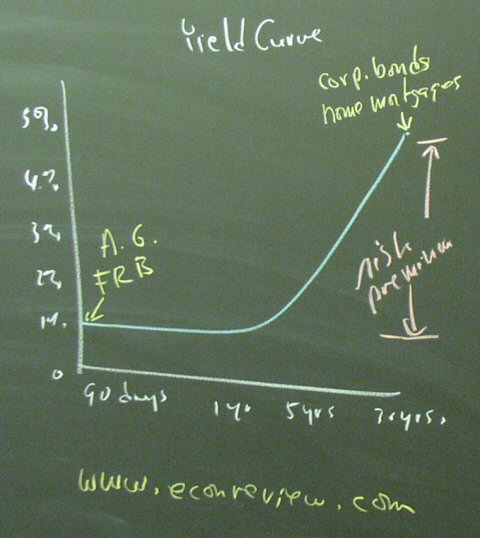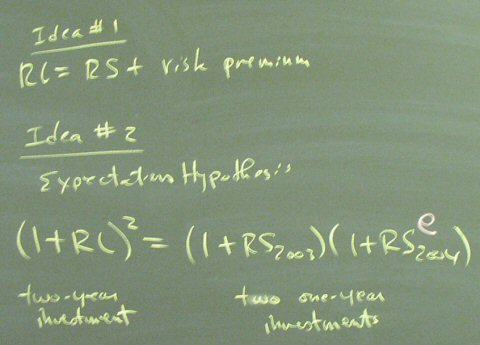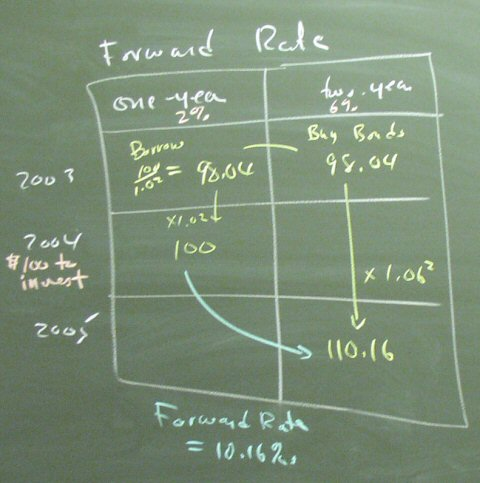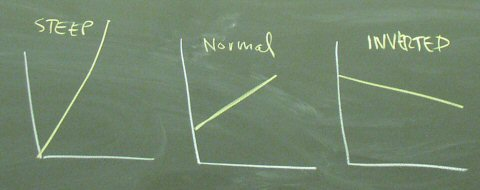## November 04, 2003

### The Term Structure of Interest Rates

The typical yield curve show higher bond yields for long-term bonds than for short-term bonds.What causes this phenomenon?We can talk about the theory of expectations. We can actually calculate the forward rate, which is essentially the empirical value of the expectation that is implicit in the data.

Calculating a forward rate:The formula for the forward rate:A brief introduction to "how to forecast using the yield curve:"Posted by bparke at November 4, 2003 12:12 PM